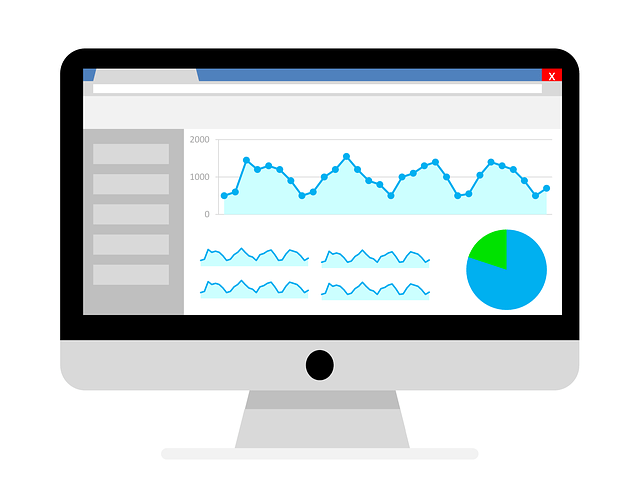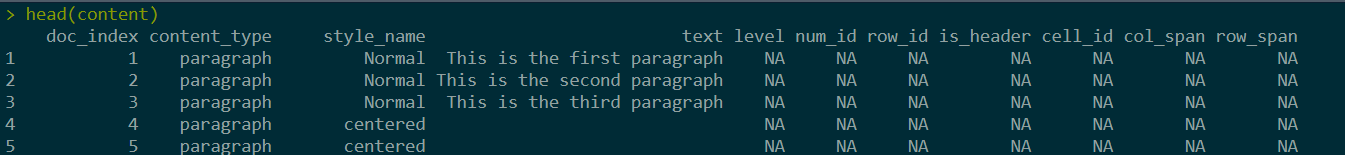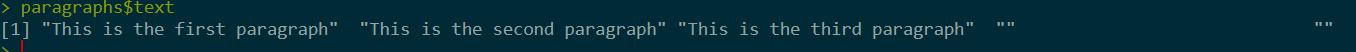Want to share your content on R-bloggers? click here if you have a blog, or here if you don't.## Reading and creating word documents in R

In this post we’ll talk about how to use R to read and create word files. We’ll primarily be using R’s officer package. For reading data from Word Documents with Python, click here.

## Creating Word reports with the officer package

The first thing we need to do is to install the officer package.

```install.packages("officer")

```

We’ll also be using the dplyr package, so you’ll need to install that the same way if you don’t have it already. Next, let’s load each of these packages.

```library(officer)
library(dplyr)

```

Now, we’ll get started creating a report! First, we will use the read_docx function to create an empty Word file.

```# create empty Word file

```

Next, let’s add a few sample paragraphs. We can do that using the body_add_par function like below. The syntax is similar to that of the tidyverse.

```sample_doc <- sample_doc %>% body_add_par("This is the first paragraph")
sample_doc <- sample_doc %>% body_add_par("This is the second paragraph")
sample_doc <- sample_doc %>% body_add_par("This is the third paragraph")

```

Now, we can add a table to our document using the body_add_table function. Before we do that, we just need to have a data frame ready, so we’ll create a sample one like below.

```# create sample data frame
df <- data.frame(a = 1:10, b = 11:20, c= 21:30)

# add table containing the data frame's contents
sample_doc <- sample_doc %>% body_add_table(df, style = "table_template")

```

### Adding images to the document

We can also add images to our Word Document. This is done by creating a temp file with an R plot and then adding the image to our document object. Though we’re using base R for plotting here, ggplot could also be used.

```set.seed(0)

# create a temp file
src <- tempfile(fileext = ".png")

# create PNG object
png(filename = src, width = 4, height = 4, units = 'in', res = 400)

# create plot
plot(sample(100, 10))

# save PNG file
dev.off()

# add PNG image to Word document
sample_doc <- sample_doc %>% body_add_img(src = src, width = 4, height = 4, style = "centered")

```

Lastly, we can save our Word Document using print.

```print(sample_doc, target = "sample_file.docx")

```

### How to modify existing Word Documents

To modify existing Word Documents, all we need to change is to input the filename into read_docx. Then, we can continue modifying our Word Document object like we were previously.

```sample_doc <- read_docx("sample_file.docx")

sample_doc <- sample_doc %>% body_add_par("This is another paragraph")

```

## How to read Word Documents with R

What if we want to read in the Word Document we just created? We can do that using the same read_docx function like we did above to modify an existing file. Secondly, we use the docx_summary with this object to get the content within the file.

```sample_data <- read_docx("sample_file.docx")

content <- docx_summary(sample_data)

```docx_summary returns a dataframe with the content in the Word file, as can be seen above. For example, to get the text in the paragraph of the document, we just need to filter the content_type field on “paragraph”, like below:

```paragraphs <- content %>% filter(content_type == "paragraph")
paragraphs\$text

```### Extracting tables from Word Documents

Now, let’s extract the table from our document. We can do this similarly to the above in that we just need to filter content_type for “table cell”:

```content %>% filter(content_type == "table cell")

```As you can see, the table’s columns are stacked in a single column. We need to do a little transformation to get this result into the needed format.

```
table_cells <- content %>% filter(content_type == "table cell")
table_data <- table_cells %>% filter(!is_header) %>% select(row_id, cell_id, text)

# split data into individual columns
splits <- split(table_data, table_data\$cell_id)
splits <- lapply(splits, function(x) x\$text)

# combine columns back together in wide format
table_result <- bind_cols(splits)

names(table_result) <- cols\$text

```## Conclusion

officer can also be used to interact with PowerPoint files, which we’ll cover in a future post. That’s all for now! Click here to follow my blog Twitter and get notified of new posts! For more on officer, check out this link.

The post How to read and create Word Documents in R appeared first on Open Source Automation.